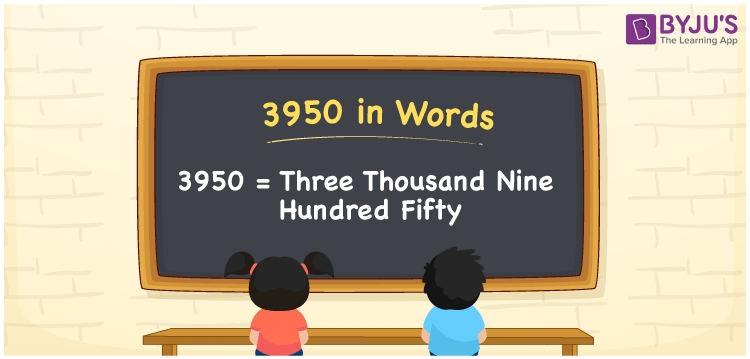# 3950 in Words

The number 3950 in words is written as Three thousand nine hundred fifty. We can quickly convert the number 3950 into words with the help of a place value chart. However, we know that the word form of 3950 is nothing but its number name. For instance, the number name for 5 is Five. Similarly, the number name for 3950 is Three thousand nine hundred fifty. Let’s learn how to derive the number 3950 in words here in this article.

 3950 in words Three thousand nine hundred fifty Three thousand nine hundred fifty in Numbers 3950

## 3950 in English Words

We generally express numbers in words using the English alphabet. So, we can spell 3950 in English as “Three thousand nine hundred fifty”.## How to Write 3950 in Words?

As we can see, 3950 is a four-digit number so we need to create a place value chart with four columns. This can be done as follows.

 Thousands Hundreds Tens Ones 3 9 5 0

Here, ones = 0, tens = 5, hundreds = 9, thousands = 3

Let us expand these digits based on their place values.

3 × Thousand + 9 × Hundred + 5 × Ten + 0 × One

= 3 × 1000 + 9 × 100 + 5 × 10 + 0 × 1

= 3000 + 900 + 50

= Three thousand + Nine hundred + Fifty

= Three thousand nine hundred fifty

Hence, 3950 in words = Three thousand nine hundred fifty.

As we know, 3950 is a natural number that precedes 3951 and succeeds 3949.

3950 in words – Three thousand nine hundred fifty

Is 3950 an odd number? – No

Is 3950 an even number? – Yes

Is 3950 a perfect square number? – No

Is 3950 a perfect cube number? – No

Is 3950 a prime number? – No

Is 3950 a composite number? – Yes

## Frequently Asked Questions on 3950 in Words

Q1

### How do you write 3950 in words?

We can write the number 3950 in words as Three thousand nine hundred fifty.
Q2

### How do you write Rs. 3950 in words on a cheque?

On a cheque, we can write Rs. 3950 in words as “Three thousand nine hundred fifty rupees only”.
Q3

### Find the value of Three thousand nine hundred fifty plus Two thousand and fifty.

Three thousand nine hundred fifty plus Two thousand and fifty = 3950 + 2050 = 6000 Hence, the value of Three thousand nine hundred fifty plus Two thousand and fifty is Six thousand.